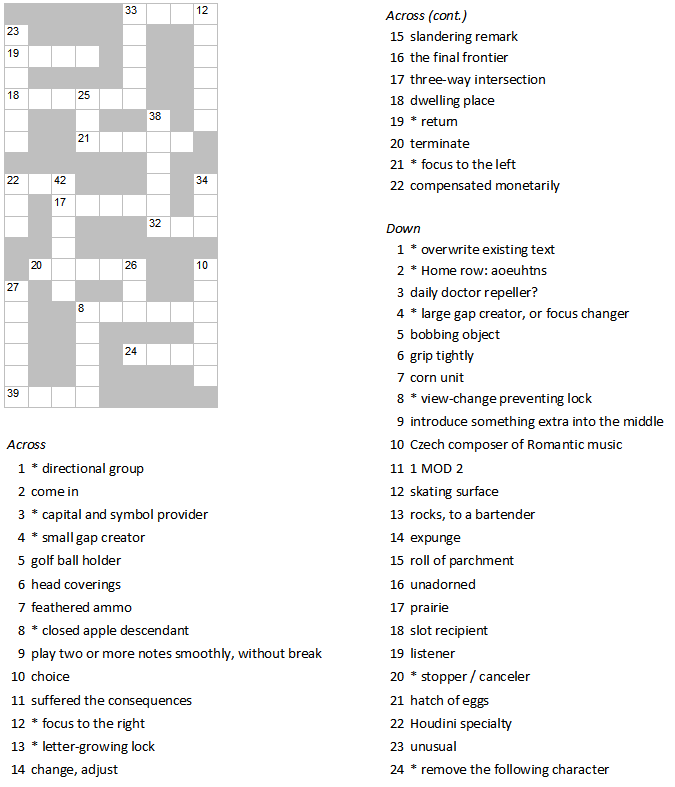All about flooble | fun stuff | Get a free chatterbox | Free JavaScript | Avatarsperplexus dot infoMixed Doubles II - Keyboard Konfusion (Posted on 2012-06-27)See Mixed Doubles.

The crossword below is special. Each answer has two clues. Your goal is to find two clues that have the same answer. Then add up their numbers, and place the answer in the appropriate box in the diagram.

For example, if the clue for 7-across is "view above (2 words)" and the clue for 21-across is "consult the textbook (2 words)", the answer for both clues would be LOOKUP. Since 7+21=28, you would fill in LOOKUP in the diagram in 28-across.

In addition, each clue that begins with * pertains to keyboards.Good luck!

 No Solution Yet Submitted by Dustin No RatingComments: ( Back to comment list | You must be logged in to post comments.)Solution Comment 1 of 1
`Across:`
`1+7=8    Arrows2+19=21  Enter3+14=17  Shift4+16=20  Space5+17=22  Tee6+13=19  Caps8+10=18  Option9+15=24  Slur11+22=33 Paid12+20=32 End18+21=39 Home`
`Down:1+9=10   Insert2+10=12  Dvorak3+5=8    Apple4+18=22  Tab6+21=27  Clutch7+19=26  Ear8+15=23  Scroll11+23=34 Odd12+13=25 Ice14+24=38 Delete16+17=33 Plain20+22=42 Escape`
`          P A I DS         L     VC A P S   A     OR         I     RO P T I O N     AL     C     D   KL     E N T E R            LT E E       E   OA   S H I F T   DB   C       E N D    A      S P A C E     IC   E     A     NL     A R R O W SU     P         ET     P   S L U RC     L         TH O M E`

 Posted by Charlie on 2012-06-27 17:13:33Please log in:

 Search: Search body:
Forums (0)